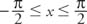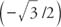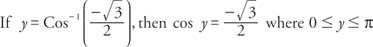## Inverse Cosine and Inverse Sine

The standard trig functions are periodic, meaning that they repeat themselves. Therefore, the same output value appears for multiple input values of the function. This makes inverse functions impossible to construct. In order to solve equations involving trig functions, it is imperative for inverse functions to exist. Thus, mathematicians have to restrict the trig function in order create these inverses.

To define an inverse function, the original function must be one‐to‐one. For a one‐to‐one correspondence to exist, (1) each value in the domain must correspond to exactly one value in the range, and (2) each value in the range must correspond to exactly one value in the domain. The first restriction is shared by all functions; the second is not. The sine function, for example, does not satisfy the second restriction, since the same value in the range corresponds to many values in the domain (see Figure  1).Figure 1
Sine function is not one to one.

To define the inverse functions for sine and cosine, the domains of these functions are restricted. The restriction that is placed on the domain values of the cosine function is 0 ≤ x ≤ π (see Figure 2 ). This restricted function is called Cosine. Note the capital “C” in Cosine.Figure 2
Graph of restricted cosine function.

The inverse cosine function is defined as the inverse of the restricted Cosine function Cos −1 (cos x) = xx ≤ π. Therefore,Figure 3
Graph of inverse cosine function.Identities for the cosine and inverse cosine:The inverse sine function's development is similar to that of the cosine. The restriction that is placed on the domain values of the sine function isThis restricted function is called Sine (see Figure  4). Note the capital “S” in Sine.Figure 4
Graph of restricted sine function.

The inverse sine function (see Figure  5) is defined as the inverse of the restricted Sine function y = Sin xFigure 5
Graph of inverse sine function.Therefore,Identities for the sine and inverse sine:The graphs of the functions y = Cos x and y = Cos −1 x are reflections of each other about the line y = x. The graphs of the functions y = Sin x and y = Sin −1 x are also reflections of each other about the line y = x (see Figure  6).Figure 6
Symmetry of inverse sine and cosine.

Example 1: Using Figure 7 , find the exact value of Cos −1.Figure 7
Drawing for Example 1.Thus, y = 5π/6 or y = 150°.

Example 2: Using Figure  8, find the exact value of Sin −1.Figure 8
Drawing for Example 2.Thus, y = π/4 or y = 45°.

Example 3: Find the exact value of cos (Cos −1 0.62).

Use the cosine‐inverse cosine identity: